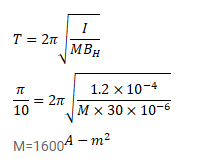# A bar magnet takes

Question:

A bar magnet takes $\pi / 10$ second to complete oscillation in an oscillation magnetometer. The moment od inertia of the magnet about the axis of rotation is $1.2 \times 10^{-4} \mathrm{~kg}-\mathrm{m}^{2}$ and the earth's horizontal magnetic field is $30^{\mu} \mathrm{T}$. Find the magnetic moment of the magnet.

Solution: